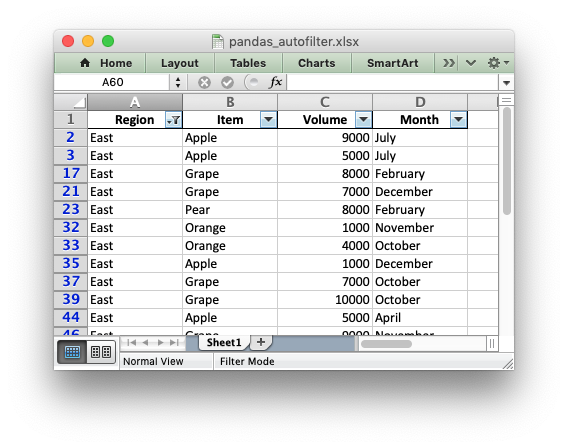# Example: Pandas Excel output with an autofilter#

An example of converting a Pandas dataframe to an Excel file with a autofilter, and filtered data, using Pandas and XlsxWriter. See Working with Autofilters for a more detailed explanation of autofilters.```##############################################################################
#
# An example of converting a Pandas dataframe to an xlsx file with an
#
# Copyright 2013-2023, John McNamara, jmcnamara@cpan.org
#

import pandas as pd

# Create a Pandas dataframe by reading some data from a space-separated file.

# Create a Pandas Excel writer using XlsxWriter as the engine.
writer = pd.ExcelWriter("pandas_autofilter.xlsx", engine="xlsxwriter")

# Convert the dataframe to an XlsxWriter Excel object. We also turn off the
# index column at the left of the output dataframe.
df.to_excel(writer, sheet_name="Sheet1", index=False)

# Get the xlsxwriter workbook and worksheet objects.
workbook = writer.book
worksheet = writer.sheets["Sheet1"]

# Get the dimensions of the dataframe.
(max_row, max_col) = df.shape

# Make the columns wider for clarity.
worksheet.set_column(0, max_col - 1, 12)

# Set the autofilter.
worksheet.autofilter(0, 0, max_row, max_col - 1)

# Add an optional filter criteria. The placeholder "Region" in the filter
# is ignored and can be any string that adds clarity to the expression.
worksheet.filter_column(0, "Region == East")

# It isn't enough to just apply the criteria. The rows that don't match
# must also be hidden. We use Pandas to figure our which rows to hide.
for row_num in df.index[(df["Region"] != "East")].tolist():
worksheet.set_row(row_num + 1, options={"hidden": True})

# Close the Pandas Excel writer and output the Excel file.
writer.close()
```